# Samacheer Kalvi 9th Maths Solutions Chapter 3 Algebra Ex 3.12

## Tamilnadu Samacheer Kalvi 9th Maths Solutions Chapter 3 Algebra Ex 3.12

Question 1.
Solve by the method of elimination
(i) 2x – y = 3; 3x + y = 7
(ii) x – y = 5; 3x + 2y = 25
(iii) $$\frac{x}{10}+\frac{y}{5}$$ = 14; $$\frac{x}{8}+\frac{y}{6}$$ = 15
(iv) 3(2x + y) = 7xy; 3(x + 3y) = 11xy
(v) $$\frac{4}{x}$$ + 5y = 7; $$\frac{3}{x}$$ + 4y = 5
(vi) 13x + 11y = 70; 11x + 13y = 74
Solution:
(i) 2x – y = 3 ………….. (1)
3x + y = 7 ………… (2)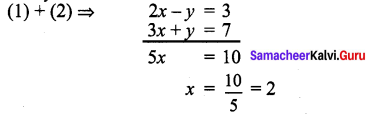Substitute x = 2 in (1)
2(2) – y = 3
4 – y = 3
-y = 3 – 4
-y = -1
∴ Solution: x = 2; y = 1
Verification:
Substitute x = 2, y = 1 in (2)
3(2) + 1 = 7 = RHS
∴ Verified.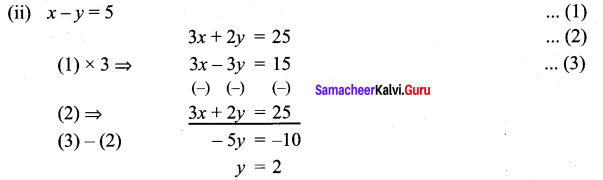Substitute y = 2 in (1)
x – 2 = 5
x = 5 + 2
x = 7
∴ Solution: x = 7, y = 2
Verification:
Substitute x = 7, y = 2 in (2)
3(7) + 2(2) = 21 + 4 = 25 = RHS
∴ Verified.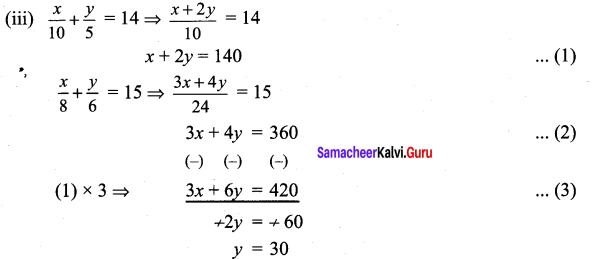Substitute y = 30 in (1)
x + 2 (30) = 140
x + 60 = 140
x = 140 – 60
x = 80
∴ Solution: x = 80; y = 30
Verification:
Substitute x = 80, y = 30 in (2)
3(80) + 4(30) = 240 + 120 = 360 = RHS
∴ Verified.

(iv) 3(2x +y) = 7xy ⇒ 6x + 3y = 7xy ………. (1)
3(x + 3y) = 11xy ⇒ 3x + 9y = 11xy ………….. (2)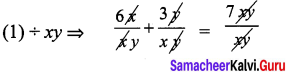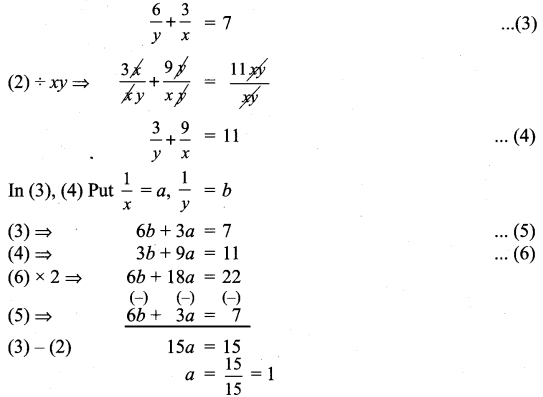Substitute a = 1 in (5)
6b + 3(1) = 7
6b + 3 = 7
6b = 7 – 3
b = $$\frac{4}{6}=\frac{2}{3}$$
∴a = $$\frac{1}{x}$$ = 1 ⇒ x = 1
b = $$\frac{1}{y}=\frac{2}{3}$$ ⇒ y = $$\frac{3}{2}$$
∴ Solution: x = 1; y = $$\frac{3}{2}$$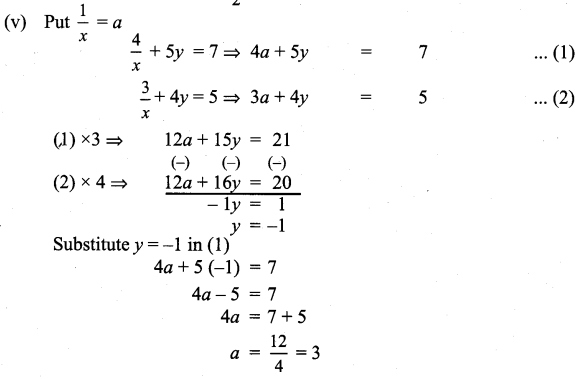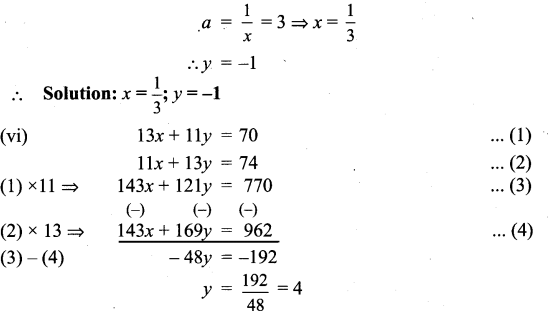Substitute y = 4 in (1)
13x + 11 (4) = 70
13x + 44 = 70
13x = 70 – 44 = 26
x = $$\frac{26}{13}$$ = 2
∴ Solution: x = 2; y = 4Question 2.
The monthly income of A and B are in the ratio 3:4 and their monthly expenditures are in the ratio 5 : 7. If each saves ₹ 5,000 per month, find the monthly income of each.
Solution:
Let the monthly income of A and B be 3x and 4x respectively.
Let the monthly expenditure of A and B be 5y and 7y respectively.
∴ 3x – 5y = 5000 ……… (1)
4x – 7y = 5000 ……….. (2)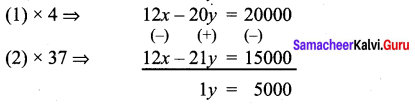Substitute y = 5000 in (1)
3x – 5 (5000) = 5000
3x – 25000 = 5000
3x = 5000 + 25000
3x = 30000
x = 10000
∴ Monthly income of A is 3x = 3 × 10000 = ₹ 30000
Monthly income of B is 4x = 4 × 10000 = ₹ 40000

Question 3.
Five years ago, a man was seven times as old as his son, while five year hence, the man will be four times as old as his son. Find their present age.
Solution:
Let the man’s present age = x
Five years ago his age is = x – 5
Let his son’s age be = y
5 years ago his son’s age = y – 5
∴ x – 5 = 7(y – 5)
x – 5 = 7y – 35
x – 7y = -35 + 5
x – 7y = – 30 ……….. (1)
After 5 years, man’s age will be = x + 5
His son’s age will be = y + 5
∴ x + 5 = 4(y + 5)
x + 5 = 4y + 20
x – 4y = 20 – 5
⇒ x – 4y = 15 ………….. (2)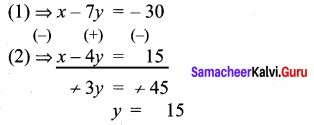Substitute y = 15 in (1)
x – 7 (15) = -30
x – 105 – 30
x = – 30 + 105
x = 75
∴ Man’s Age = 75, His son’s Age =15